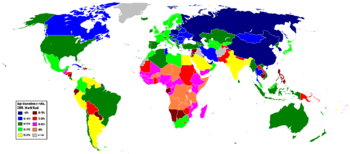# Dependency ratio

﻿
Dependency ratioAge dependency ratio by country, 2008. Based on World Bank data.

In economics and geography the dependency ratio is an age-population ratio of those typically not in the labor force (the dependent part) and those typically in the labor force (the productive part). It is used to measure the pressure on productive population

## Formula

In published international statistics, the dependent part usually includes those under the age of 15 and over the age of 64. The productive part makes up the population in between, ages 15 – 64. It is normally expressed as a percentage:$(Total)\ Dependency\ ratio = \frac{(number\ of\ people\ aged\ 0-14\ and\ those\ aged\ 65\ and\ over)} {number\ of\ people\ aged\ 15-64} \times 100$

As the ratio increases there may be an increased burden on the productive part of the population to maintain the upbringing and pensions of the economically dependent. This results in direct impacts on financial expenditures on things like social security, as well as many indirect consequences.

The (total) dependency ratio can be decomposed into the child dependency ratio and the aged dependency ratio:$Child\ dependency\ ratio = \frac{number\ of\ people\ aged\ 0-14} {number\ of\ people\ aged\ 15-64} \times 100$$Aged\ dependency\ ratio\ = \frac{number\ of\ people\ aged\ 65\ and\ over} {number\ of\ people\ aged\ 15-64} \times 100$

## Inverse

The inverse of the dependency ratio, the inverse dependency ratio can be interpreted as how many independent workers have to provide for one dependent person (pension & expenditure on children)

## Problems

A high dependency ratio can cause serious problems for a country. As the largest proportion of a government's expenditure is on health, social security & education which are most used by old and young population. Also the increasing expenditure on pension becomes a problem too.

## Migrant Labor Dependency Ratio

Migrant Labor Dependency Ratio (MLDR) is used to describe the extent to which the domestic population is dependent upon migrant labor.